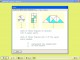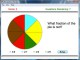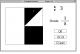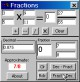Fraction Fusion 2.1 Fraction Fusion teaches and reviews fraction concepts to middle elementary-aged students. DemoFraction Shape-Up 1.00 Interactive software that helps students understand and work with fractions. SharewareFractionAction 0.9 FractionAction will do the following: reduce fractions; fraction arithmetic; decimal to/from fraction conversions with periodic (repeating) digits displayed; fraction to/from continued fraction conversions for common fractions and fractions with simple square roots. SharewareFraction Fonts 2.0 Fraction Fonts are a handy way for Mac-based Desktop Publishers to add the most commonly used fractions to their documents. DemoFraction Squares 1.0 Fraction Squares shows a series of 160 pre-set drawings. FreewareAbilities Builder Fraction Facts 3.5 Build fraction reducing and simplifying skills for simple to complex problems. DemoFraction Plugin 1.0 Fraction Plugin is a plugin for REALbasic 5 which, because of operator and function overloading, allows fractions to be used much like doubles and integers in REALbasic programs. FreewareFRS Fraction Master 2.1 Learn fractions faster. SharewareFraction Puzzles 1.0 Middle-School (grades 5 through 9) math program written to provide skills in context. FreewareFractions n Decimals CE 5.3 Add, Subtract, Divide and Multiply fractions. Convert decimals/fractions CE 2.11 Shareware